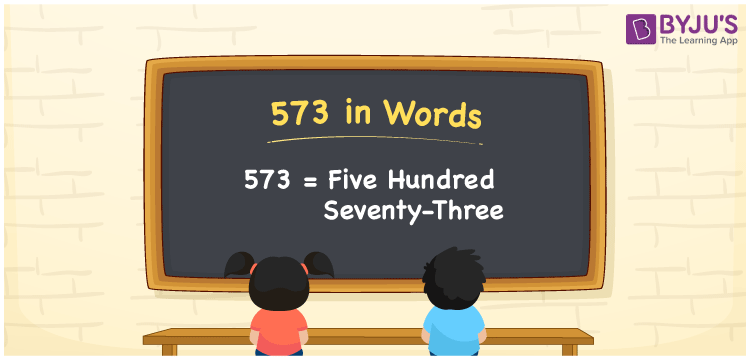# 573 in words

573 in words is written as Five Hundred and Seventy Three. 573 represents the count or value. The article on Counting Numbers can give you an idea about count or counting. The number 573 is used in expressions that relate to money, distance, length, year and others. Let us consider an example for 573. ”I had to write an article with only Five hundred words, but I ended up writing Five Hundred and Seventy Three words.”

 573 in words Five Hundred and Seventy Three Five Hundred and Seventy Three in Numbers 573

## 573 in English Words## How to Write 573 in Words?

We can convert 573 to words using a place value chart. The number 573 has 3 digits, so let’s make a chart that shows the place value up to 3 digits.

 Hundreds Tens Ones 5 7 3

Thus, we can write the expanded form as:

= 5 × Hundred + 7 × Ten + 3 × One

= 5 × 100 + 7 × 10 + 3 × 1

= 573

= Five Hundred and Seventy Three.

573 is the natural number that is succeeded by 572 and preceded by 574.

573 in words – Five Hundred and Seventy Three.

Is 573 an odd number? – Yes.

Is 573 an even number? – No.

Is 573 a perfect square number? – No.

Is 573 a perfect cube number? – No.

Is 573 a prime number? – No.

Is 573 a composite number? – Yes.

## Solved Example

1. Write the number 573 in expanded form

Solution: 5 × 100 + 7 × 10 + 3 × 1

We can write 573 = 500 + 70 + 3

= 5 × 100 + 7 × 10 + 3 × 1

## Frequently Asked Questions on 573 in words

Q1

### How to write 573 in words?

573 in words is written as Five Hundred and Seventy Three.
Q2

### Is 573 a perfect square number?

No. 573 is not a perfect square number.
Q3

### Is 573 a prime number?

No. 573 is not a prime number.